# Probability Aggregation Methods in Geoscience

## Abstract

The need for combining different sources of information in a probabilistic framework is a frequent task in earth sciences. This is a need that can be seen when modeling a reservoir using direct geological observations, geophysics, remote sensing, training images, and more. The probability of occurrence of a certain lithofacies at a certain location for example can easily be computed conditionally on the values observed at each source of information. The problem of aggregating these different conditional probability distributions into a single conditional distribution arises as an approximation to the inaccessible genuine conditional probability given all information. This paper makes a formal review of most aggregation methods proposed so far in the literature with a particular focus on their mathematical properties. Exact relationships relating the different methods is emphasized. The case of events with more than two possible outcomes, never explicitly studied in the literature, is treated in detail. It is shown that in this case, equivalence between different aggregation formulas is lost. The concepts of calibration, sharpness, and reliability, well known in the weather forecasting community for assessing the goodness-of-fit of the aggregation formulas, and a maximum likelihood estimation of the aggregation parameters are introduced. We then prove that parameters of calibrated log-linear pooling formulas are a solution of the maximum likelihood estimation equations. These results are illustrated on simulations from two common stochastic models for earth science: the truncated Gaussian model and the Boolean. It is found that the log-linear pooling provides the best prediction while the linear pooling provides the worst.

This is a preview of subscription content, access via your institution.

## References

• Allard D, D’Or D, Froidevaux R (2011) An efficient maximum entropy approach for categorical variable prediction. Eur J Soil Sci 62(3):381–393

• Bacharach M (1979) Normal Bayesian dialogues. J Am Stat Assoc 74:837–846

• Benediktsson J, Swain P (1992) Consensus theoretic classification methods. IEEE Trans Syst Man Cybern 22:688–704

• Bordley RF (1982) A multiplicative formula for aggregating probability assessments. Manag Sci 28:1137–1148

• Brier G (1950) Verification of forecasts expressed in terms of probability. Mon Weather Rev 78:1–3

• Bröcker J, Smith LA (2007) Increasing the reliability of reliability diagrams. Weather Forecast 22:651–661

• Cao G, Kyriakidis P, Goodchild M (2009) Prediction and simulation in categorical fields: a transition probability combination approach. In: Proceedings of the 17th ACM SIGSPATIAL international conference on advances in geographic information systems, GIS’09. ACM, New York, pp 496–499

• Christakos G (1990) A Bayesian/maximum-entropy view to the spatial estimation problem. Math Geol 22:763–777

• Chugunova T, Hu L (2008) An assessment of the tau model for integrating auxiliary information. In: Ortiz JM, Emery X (eds) VIII international geostatistics congress, Geostats 2008. Gecamin, Santiago, pp 339–348

• Clemen RT, Winkler RL (1999) Combining probability distributions from experts in risk analysis. Risk Anal 19:187–203

• Clemen RT, Winkler W (2007) Aggregating probability distributions. In: Edwards W, Miles RF, von Winterfeldt D (eds) Advances in decision analysis. Cambridge University Press, Cambridge, pp 154–176

• Comunian A (2010) Probability aggregation methods and multiple-point statistics for 3D modeling of aquifer heterogeneity from 2D training images. PhD thesis, University of Neuchâtel, Switzerland

• Comunian A, Renard P, Straubhaar J (2011) 3D multiple-point statistics simulation using 2D training images. Comput Geosci 40:49–65

• Cover TM, Thomas JA (2006) Elements of information theory, 2nd edn. Wiley, New York

• Dietrich F (2010) Bayesian group belief. Soc Choice Welf 35:595–626

• Genest C (1984) Pooling operators with the marginalization property. Can J Stat 12:153–165

• Genest C, Wagner CG (1987) Further evidence against independence preservation in expert judgement synthesis. Aequ Math 32:74–86

• Genest C, Zidek JV (1986) Combining probability distributions: a critique and an annotated bibliography. Stat Sci 1:114–148

• Gneiting T, Raftery AE (2007) Strictly proper scoring rules, prediction, and estimation. J Am Stat Assoc 102:359–378

• Heskes T (1998) Selecting weighting factors in logarithmic opinion pools. In: Jordan M, Kearns M, Solla S (eds) Advances in neural information processing systems, vol 10. MIT Press, Cambridge, pp 266–272

• Journel A (2002) Combining knowledge from diverse sources: an alternative to traditional data independence hypotheses. Math Geol 34:573–596

• Krishnan S (2008) The Tau model for data redundancy and information combination in earth sciences: theory and application. Math Geosci 40:705–727

• Kullback S, Leibler RA (1951) On information and sufficiency. Ann Math Stat 22:76–86

• Lantuéjoul C (2002) Geostatistical simulations. Springer, Berlin

• Lehrer K, Wagner C (1983) Probability amalgamation and the independence issue: a reply to Laddaga. Synthese 55:339–346

• Mariethoz G, Renard P, Froidevaux R (2009) Integrating collocated auxiliary parameters in geostatistical simulations using joint probability distributions and probability aggregation. Water Resour Res 45(W08421):1–13

• Okabe H, Blunt MJ (2004) Prediction of permeability for porous media reconstructed using multiple-point statistics. Phys Rev E 70(6):066135

• Okabe H, Blunt MJ (2007) Pore space reconstruction of vuggy carbonates using microtomography and multiple-point statistics. Water Resour Res 43(W12S02):1–5

• Polyakova EI, Journel AG (2007) The nu expression for probabilistic data integration. Math Geol 39:715–733

• Ranjan R, Gneiting T (2010) Combining probability forecasts. J R Stat Soc B 72:71–91

• Schwartz G (1978) Estimating the dimension of a model. Ann Stat 6:461–464

• Stone M (1961) The opinion pool. Ann Math Stat 32:1339–1348

• Strebelle S, Payrazyan K, Caers J (2003) Modeling of a deepwater turbidite reservoir conditional to seismic data using principal component analysis and multiple-point geostatistics. SPE J 8:227–235

• Tarantola A (2005) Inverse problem theory. Society for Industrial and Applied Mathematics, Philadelphia

• Tarantola A, Valette B (1982) Inverse problems = quest for information. J Geophys 50:159–170

• Wagner C (1984) Aggregating subjective probabilities: some limitative theorems. Notre Dame J Form Log 25:233–240

• Winkler RL (1968) The consensus of subjective probability distributions. Manag Sci 15:B61–B75

## Acknowledgements

Funding for A. Comunian and P. Renard was mainly provided by the Swiss National Science foundation (Grants PP002-106557 and PP002-124979) and the Swiss Confederation’s Innovation Promotion Agency (CTI Project No. 8836.1 PFES-ES) A. Comunian was partially supported by the Australian Research Council and the National Water Commission.

## Author information

Authors

### Corresponding author

Correspondence to D. Allard.

The order of the authors is alphabetical.

## Appendices

### Appendix A: Maximum Entropy

Let us define Q(A,D 0,D 1,…,D n ) the joint probability distribution maximizing its entropy $$H(Q) = -\sum_{A \in{\mathcal{A}}} Q(D_{0},D_{1},\dots,D_{n})(A) \ln Q(D_{0},D_{1},\dots,D_{n}) (A)$$ subject to the following constraints.

1. 1.

Q(A,D 0)=Q(AD 0)Q(D 0)∝P 0(A), for all $$A \in {\mathcal{A}}$$.

2. 2.

Q(A,D 0,D i )=Q(AD i )Q(D i )Q(D 0)∝P i (A), for all $$A \in{\mathcal{A}}$$ and all i=1,…,n.

We will first show that

$$Q(A,D_0,D_1,\dots,D_n) \propto P_0(A)^{1-n} \prod_{i=1}^{n}P_i(A),$$

from which the conditional probability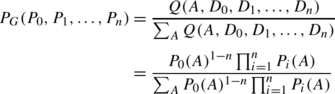is immediately derived. For ease of notation, we will use ∑ A as a short notation for $$\sum_{A \in{\mathcal{A}}}$$.

### Proof

The adequate approach is to use the Lagrange multiplier technique on the objective function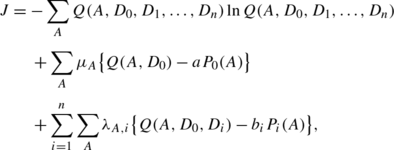where μ A and λ A,i are Lagrange multipliers. For finding the solution Q optimizing the constrained problem, we set all partial derivatives to 0. This leads to the system of equations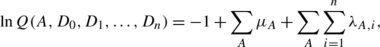(54)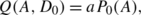(55)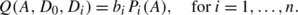(56)

From Eqs. (54) and (55), we get

$$Q(A,D_0) = e^{-1}\prod_A e^{\mu_A} \propto P_0(A).$$

Similarly, from Eqs. (54) and (56), we get

$$Q(A,D_0,D_i) = Q(A,D_0) \prod_A e^{\lambda_{A,i}} \propto P_i(A),\quad \hbox{for}\ i=1,\dots,n,$$

from which we find

$$\prod_A e^{\lambda_{A,i}} \propto P_i(A)/P_0(A),\quad \hbox{for}\ i=1,\dots,n.$$

Plugging this in Eq. (54) yields

$$Q(A,D_0,D_1,\dots,D_n) \propto P_0(A) \prod_{i=1}^n \frac{P_i(A)}{P_0(A)}.$$

Hence,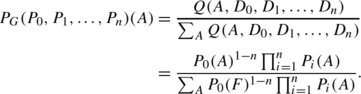□

### Appendix B: Conditional Probabilities for the Trinary Event Example

1. Let us first compute the conditional probability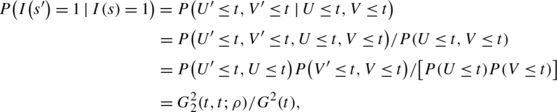where $$G^{2}_{2}(t,t;\rho)$$ is the bivariate cpf of a (0,1) bi-Gaussian random vector with correlation ρ. For symmetry reasons, one has P(I(s′)=2∣I(s)=1)=P(I(s′)=3∣I(s)=1), from which it follows immediately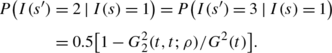2. We consider now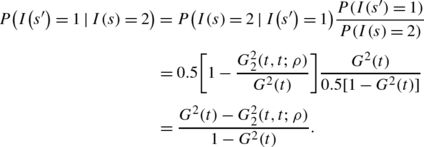3. The picture is slightly more complicated for P(I(s′)=2∣I(s)=2)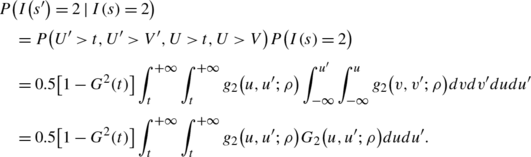There is no closed-form expression for the double integral which must be evaluated numerically. Then P(I(s′)=3∣I(s)=2) is computed as the complement to 1.

4. The conditional probabilities of I(s′) given that I(s)=3 are then obtained by symmetry.

## Rights and permissions

Reprints and Permissions

Allard, D., Comunian, A. & Renard, P. Probability Aggregation Methods in Geoscience. Math Geosci 44, 545–581 (2012). https://doi.org/10.1007/s11004-012-9396-3

• Accepted:

• Published:

• Issue Date:

• DOI: https://doi.org/10.1007/s11004-012-9396-3

### Keywords

• Data integration
• Conditional probability pooling
• Calibration
• Sharpness
• Log-linear pooling